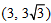# Let P = (–1, 0), Q = (0, 0) and R =be three points. What is the equation of the bisector of the angle PQR?

A.
B.
C.
D.
Anonymous User Maths Two Dimensional Geometry 24 Jul, 2020 57 views